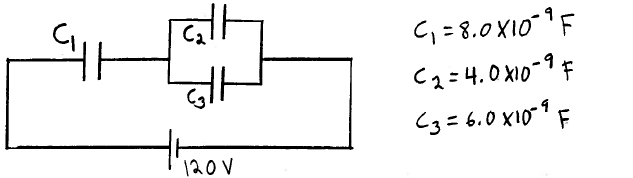Physics Practice Problems Solving Capacitor Circuits Practice Problems Solution: Consider the circuit shown in the sketch. When the...

# Solution: Consider the circuit shown in the sketch. When the capacitors have their final charges, what is the charge on C2?

###### Problem

Consider the circuit shown in the sketch. When the capacitors have their final charges, what is the charge on C2?Solving Capacitor Circuits

Solving Capacitor Circuits

#### Q. Three capacitors C1 = 4µF, C2 = 8µF, and C3 = 6µF are connected across a 20V battery as shown. Find the voltage drop across C1. Find the voltage d...

Solved • Sun Jun 18 2017 23:01:46 GMT-0400 (EDT)

Solving Capacitor Circuits

#### Q. Three capacitors C1 = 4μF, C2 =8μF, and C3 = 6μF are connected across a 20 V battery as shown. a) Find the voltage drop across C1. b) Find the volta...

Solved • Tue May 30 2017 15:15:54 GMT-0400 (EDT)

Solving Capacitor Circuits

#### Q. Find the charge out of the 10V battery in the circuit? (a) 2.5 μC (b) 48 μC (c) 72 μC (d) 96 μC

Solved • Mon May 29 2017 15:16:59 GMT-0400 (EDT)

Solving Capacitor Circuits

#### Q. Three capacitors are connected to a 1 V battery as shown beloe. The capacitances are C1 = 3 F, C2 = 1 F and C3 = 5 F. Rank the capacitors according to...

Solved • Thu Apr 27 2017 14:18:25 GMT-0400 (EDT)